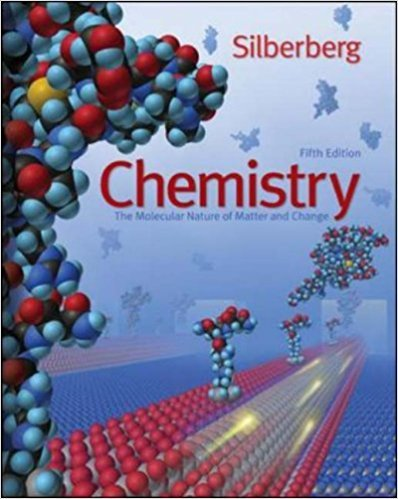×
×

# Solutions for Chapter 3: Stoichiometry of Formulas and Equations## Full solutions for Chemistry: The Molecular Nature of Matter and Change | 5th Edition

ISBN: 9780077216504Solutions for Chapter 3: Stoichiometry of Formulas and Equations

Solutions for Chapter 3
4 5 0 408 Reviews
19
5
##### ISBN: 9780077216504

Chemistry: The Molecular Nature of Matter and Change was written by and is associated to the ISBN: 9780077216504. This expansive textbook survival guide covers the following chapters and their solutions. Chapter 3: Stoichiometry of Formulas and Equations includes 62 full step-by-step solutions. Since 62 problems in chapter 3: Stoichiometry of Formulas and Equations have been answered, more than 79047 students have viewed full step-by-step solutions from this chapter. This textbook survival guide was created for the textbook: Chemistry: The Molecular Nature of Matter and Change, edition: 5.

Key Chemistry Terms and definitions covered in this textbook
• blocking group

A group that can be readily installed and uninstalled. Used for regiochemical control during synthesis.

• bond length

The distance between the centers of two bonded atoms. (Section 8.3)

• chelate effect

The generally larger formation constants for polydentate ligands as compared with the corresponding monodentate ligands. (Section 23.3)

• coordination compound

A compound containing a metal ion bonded to a group of surrounding molecules or ions that act as ligands. (Section 23.2)

• coordination sphere

The metal ion and its surrounding ligands. (Section 23.2)

• electron capture

A mode of radioactive decay in which an inner-shell orbital electron is captured by the nucleus. (Section 21.1)

• functional group

A characteristic group of atoms/bonds that possess a predictable chemical behavior.

• gray (Gy)

The SI unit for radiation dose

• Hertz (Hz)

The unit in which frequency is measured: s 21 (read “per second”).

• Heterocycle

A cyclic compound whose ring contains more than one kind of atom. Oxirane (ethylene oxide), for example, is a heterocycle whose ring contains two carbon atoms and one oxygen atom.

• Hydride ion

A hydrogen atom with two electrons in its valence shell; H:!

• hydrophobic

Water repelling. The term is often used to describe a type of colloid. (Section 13.6)

• Line-angle formula

An abbreviated way to draw structural formulas in which vertices and line endings represent carbons

• Merrifield synthesis

A method for building a peptide from protected building blocks.

• organic chemistry

The study of carbon-containing compounds, typically containing carbon–carbon bonds. (Section 2.9; Chapter 24:Introduction)

• pyrimidine

A compound that is similar in structure to pyridine but contains one extra nitrogen atom at the 3 position.

• specific heat 1Cs2

The heat capacity of 1 g of a substance; the heat required to raise the temperature of 1 g of a substance by 1 °C. (Section 5.5)

• thermosetting resins

Highly crosslinked polymers that are generally very hard and insoluble.

• three-center, two-electron bonds

A bond in which two electrons are associated with three atoms, such as in diborane (B2H6).

• Williamson ether synthesis

A general method for the synthesis of dialkyl ethers by an SN2 reaction between a haloalkane and an alkoxide ion.

×